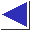# comp.lang.c FAQ list ·Question 15.4

Q: How can I write a function that takes a variable number of arguments?

A: Use the facilities of the <stdarg.h> header.

Here is a function which concatenates an arbitrary number of strings into malloc'ed memory:

```#include <stdlib.h>		/* for malloc, NULL, size_t */
#include <stdarg.h>		/* for va_ stuff */
#include <string.h>		/* for strcat et al. */

char *vstrcat(const char *first, ...)
{
size_t len;
char *retbuf;
va_list argp;
char *p;

if(first == NULL)
return NULL;

len = strlen(first);

va_start(argp, first);

while((p = va_arg(argp, char *)) != NULL)
len += strlen(p);

va_end(argp);

retbuf = malloc(len + 1);	/* +1 for trailing \0 */

if(retbuf == NULL)
return NULL;		/* error */

(void)strcpy(retbuf, first);

va_start(argp, first);		/* restart; 2nd scan */

while((p = va_arg(argp, char *)) != NULL)
(void)strcat(retbuf, p);

va_end(argp);

return retbuf;
}
```
(Note that a second call to va_start is needed to re-start the scan when the argument list is processed a second time. Note the calls to va_end: they're important for portability, even if they don't seem to do anything.)

A call to vstrcat looks something like

```	char *str = vstrcat("Hello, ", "world!", (char *)NULL);
```
Note the cast on the last argument; see questions 5.2 and 15.3. (Also note that the caller must free the returned, malloc'ed storage.)

vstrcat accepts a variable number of arguments, all of type char *. Here is an example which accepts a variable number of arguments of different types; it is a stripped-down version of the familiar printf function. Note that each invocation of va_arg() specifies the type of the argument being retrieved from the argument list.

(The miniprintf function here uses baseconv from question 20.10 to format numbers. It is significantly imperfect in that it will not usually be able to print the smallest integer, INT_MIN, properly.)

```#include <stdio.h>
#include <stdarg.h>
#ifdef MAIN

void miniprintf(const char *, ...);

main()
{
miniprintf("Hello, world!\n");
miniprintf("%c %d %s\n", '1', 2, "three");
miniprintf("%o %d %x\n", 10, 10, 10);
miniprintf("%u\n", 0xffff);
return 0;
}

#endif

extern char *baseconv(unsigned int, int);

void
miniprintf(const char *fmt, ...)
{
const char *p;
int i;
unsigned u;
char *s;
va_list argp;

va_start(argp, fmt);

for(p = fmt; *p != '\0'; p++) {
if(*p != '%') {
putchar(*p);
continue;
}

switch(*++p) {
case 'c':
i = va_arg(argp, int);
/* *not* va_arg(argp, char); see Q 15.10 */
putchar(i);
break;

case 'd':
i = va_arg(argp, int);
if(i < 0) {
/* XXX won't handle INT_MIN */
i = -i;
putchar('-');
}
fputs(baseconv(i, 10), stdout);
break;

case 'o':
u = va_arg(argp, unsigned int);
fputs(baseconv(u, 8), stdout);
break;

case 's':
s = va_arg(argp, char *);
fputs(s, stdout);
break;

case 'u':
u = va_arg(argp, unsigned int);
fputs(baseconv(u, 10), stdout);
break;

case 'x':
u = va_arg(argp, unsigned int);
fputs(baseconv(u, 16), stdout);
break;

case '%':
putchar('%');
break;
}
}

va_end(argp);
}
```